This document contains R versions of the boxed examples from Chapter 10 of the “Analysis and Interpretation of Freshwater Fisheries Data” book. Some sections build on descriptions from previous sections, so each section may not stand completely on its own. More thorough discussions of the following items are available in linked vignettes:

The following additional packages are required to complete all of the examples (with the required functions noted as a comment and also noted in the specific examples below).

``````> library(FSA)          # Summarize, fitPlot, residPlot, addSigLetters, lencat, headtail
> library(car)          # Anova
> library(multcomp)     # glht, mcp
> library(lattice)      # xyplot``````

In addition, external tab-delimited text files are used to hold the data required for each example. These data are loaded into R in each example with `read.table()`. Before using `read.table()` the working directory of R must be set to where these files are located on your computer. The working directory for all data files on my computer is set with

``> setwd("C:/aaaWork/Web/fishR/BookVignettes/aiffd2007")``

In addition, I prefer to not show significance stars for hypothesis test output, reduce the margins on plots, alter the axis label positions, make all axis tick labels horizontal, and reduce the axis tick length. In addition, contrasts are set in such a manner as to force R output to match SAS output for linear model summaries. All of these options are set with

``````> options(width=90,continue=" ",show.signif.stars=FALSE,
contrasts=c("contr.sum","contr.poly"))
> par(mar=c(3.5,3.5,0.5,0.5),mgp=c(2.1,0.4,0),las=1,tcl=-0.2)``````

## 10.1 Transformation and Regression Analyses of Weight-Length Data – Comparing the Condition of Two Populations

Data collected are total length (`TL`; mm), weight (`WT`;g), and body fat as a percentage of overall wet weight for samples of Yellowstone Cutthroat Trout (Oncorhynchus clarkia) collected in midsummer from three locations that could influence individual weight at length: a lower-elevation stream (1,810 m elevation), a lower-elevation lake (1,785 m), and a higher-elevation lake (2,610 m). Fat values were randomly generated for example only. Fish samples were collected via electroshocking, gill nets, and angling.

### 10.1.1 Preparing Data

The Box10_1.txt data file is read, the structure of the data frame is observed, and common log transformations of the total length (`TL`) and weight (`WT`) variables are appended to the data frame.

``````> d1 <- read.table("data/Box10_1.txt",header=TRUE)
> str(d1)``````
``````'data.frame':   150 obs. of  4 variables:
\$ POP: Factor w/ 3 levels "A","B","C": 1 1 1 1 1 1 1 1 1 1 ...
\$ TL : int  129 130 132 132 134 134 138 140 143 144 ...
\$ WT : int  20 25 22 24 20 25 26 28 28 30 ...
\$ FAT: num  5.91 12.88 7.67 11.29 2.27 ...``````
``````> d1\$logTL <- log10(d1\$TL)
> d1\$logWT <- log10(d1\$WT)
> str(d1)``````
``````'data.frame':   150 obs. of  6 variables:
\$ POP  : Factor w/ 3 levels "A","B","C": 1 1 1 1 1 1 1 1 1 1 ...
\$ TL   : int  129 130 132 132 134 134 138 140 143 144 ...
\$ WT   : int  20 25 22 24 20 25 26 28 28 30 ...
\$ FAT  : num  5.91 12.88 7.67 11.29 2.27 ...
\$ logTL: num  2.11 2.11 2.12 2.12 2.13 ...
\$ logWT: num  1.3 1.4 1.34 1.38 1.3 ...``````

In the box the authors perform separate analyses for the Cutthroat Trout from “population A” and “population B.” To facilitate these analyses, I created two new data frames using `Subset()` (from the `FSA` package) that will separately contain just the fish from these two populations. The `Subset()` function requires two arguments. The first argument is the data frame from which the subset should be extracted. The second argument is a “conditioning statement” that explains how the subset should be constructed. The structure for each data frame shows that the number of levels for the `POP` variable is only one, indicating that the subsetting was successful.

``````> d1A <- Subset(d1,POP=="A")
> str(d1A)``````
``````'data.frame':   50 obs. of  6 variables:
\$ POP  : Factor w/ 1 level "A": 1 1 1 1 1 1 1 1 1 1 ...
\$ TL   : int  129 130 132 132 134 134 138 140 143 144 ...
\$ WT   : int  20 25 22 24 20 25 26 28 28 30 ...
\$ FAT  : num  5.91 12.88 7.67 11.29 2.27 ...
\$ logTL: num  2.11 2.11 2.12 2.12 2.13 ...
\$ logWT: num  1.3 1.4 1.34 1.38 1.3 ...``````
``````> d1B <- Subset(d1,POP=="B")
> str(d1B)``````
``````'data.frame':   50 obs. of  6 variables:
\$ POP  : Factor w/ 1 level "B": 1 1 1 1 1 1 1 1 1 1 ...
\$ TL   : int  254 262 272 274 282 287 290 297 302 305 ...
\$ WT   : int  181 186 136 191 245 236 168 263 290 290 ...
\$ FAT  : num  10.59 9.08 1.38 2.64 7.32 ...
\$ logTL: num  2.4 2.42 2.43 2.44 2.45 ...
\$ logWT: num  2.26 2.27 2.13 2.28 2.39 ...``````

### 10.1.2 Linear Regression of the Transformed Data

The linear regression model is fit in R with `lm()` with a model formula of the form `response`~`explanatory` and a `data=` argument where the variables are contained. The ANOVA table, coefficients summary table, and confidence intervals for the coefficients are extracted by submitting the saved `lm` object to `anova()`, `summary()` (or `coef()`), and `confint()`, respectively.

``````> lm1 <- lm(logWT~logTL,data=d1A)
> anova(lm1)``````
``````          Df Sum Sq Mean Sq F value    Pr(>F)
logTL      1 8.5427  8.5427  4545.7 < 2.2e-16
Residuals 48 0.0902  0.0019
Total     49 8.6329                          ``````
``> summary(lm1)``
``````Coefficients:
Estimate Std. Error t value Pr(>|t|)
(Intercept) -5.14432    0.10630  -48.39   <2e-16
logTL        3.06874    0.04552   67.42   <2e-16

Residual standard error: 0.04335 on 48 degrees of freedom
Multiple R-squared: 0.9896, Adjusted R-squared: 0.9893
F-statistic:  4546 on 1 and 48 DF,  p-value: < 2.2e-16 ``````
``> confint(lm1)``
``````                2.5 %    97.5 %
(Intercept) -5.358049 -4.930587
logTL        2.977220  3.160250``````

A summary graphic of the regression model fit is obtained with `fitPlot()` (from the `FSA` package) which requires the saved `lm` object as its first argument. In addition, the x- and y-axes can be labeled as usual and the default main graphic label can be suppressed by including `main=""`. A residual plot for assessing linearity and homoscedasticity is constructed with `residPlot()` (from the `FSA` package), again submitting only the saved `lm` object as an argument.

``> fitPlot(lm1,xlab="log10(Total Length)",ylab="log10(Weight)",main="")``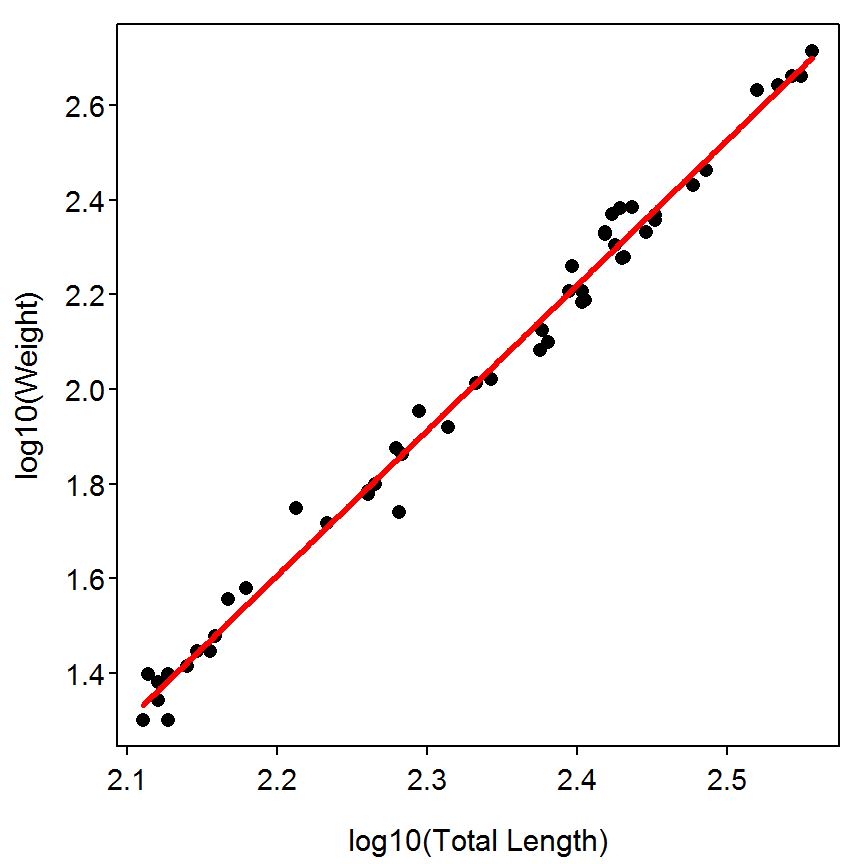``> residPlot(lm1)``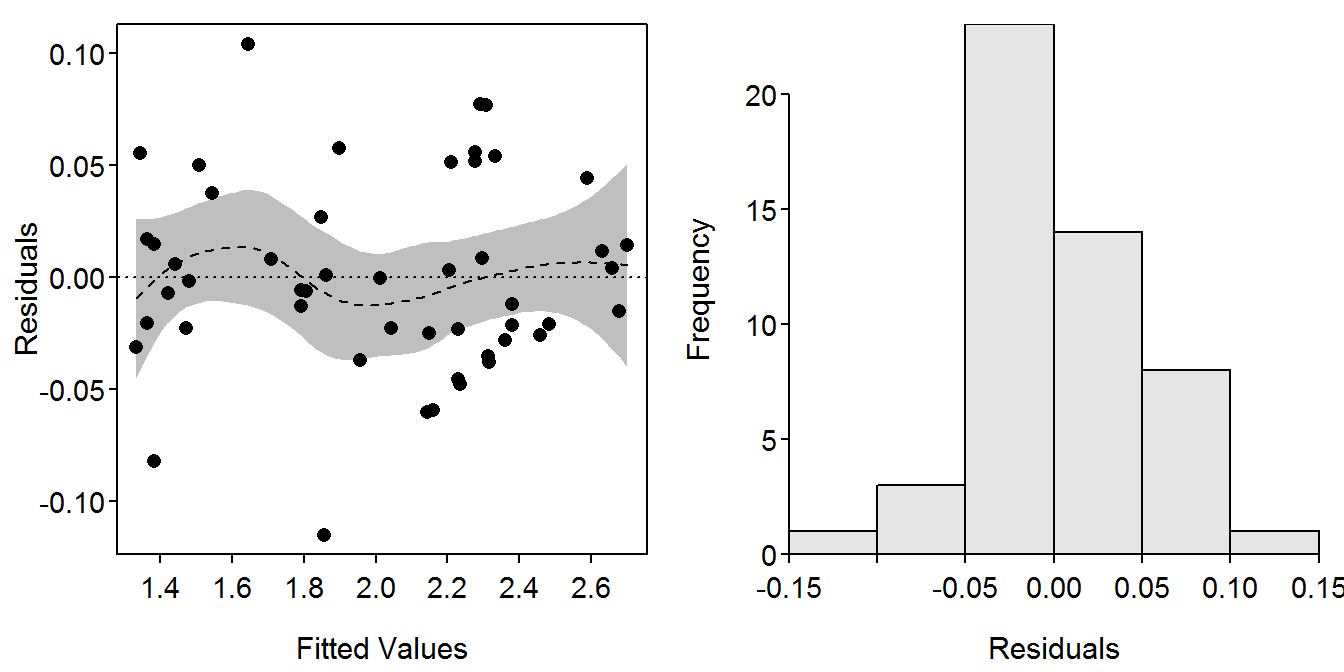A similar analysis can be easily constructed for “population B” (note change in `data=`.

``````> lm2 <- lm(logWT~logTL,data=d1B)
> anova(lm2)``````
``````          Df  Sum Sq Mean Sq F value    Pr(>F)
logTL      1 1.66271 1.66271  914.86 < 2.2e-16
Residuals 48 0.08724 0.00182
Total     49 1.74995                          ``````
``> summary(lm2)``
``````Coefficients:
Estimate Std. Error t value Pr(>|t|)
(Intercept)  -5.3694     0.2646  -20.29   <2e-16
logTL         3.1431     0.1039   30.25   <2e-16

Residual standard error: 0.04263 on 48 degrees of freedom
Multiple R-squared: 0.9501, Adjusted R-squared: 0.9491
F-statistic: 914.9 on 1 and 48 DF,  p-value: < 2.2e-16 ``````
``> confint(lm2)``
``````                2.5 %    97.5 %
(Intercept) -5.901353 -4.837372
logTL        2.934132  3.352001``````

The text in the box suggests that the authors are interested in predicting the mean weights of 250, 300, and 450 mm trout from each population. These calculations are constructed with `predict()`. This function requires the saved linear model as the first object and a data frame of values of the explanatory variable at which predictions of the response variable should be made as the second argument. Finally, a confidence interval for the mean can be constructed by including the `interval="c"` argument or a prediction interval for an individual can be constructed with the `interval="p"` argument. In this example, it is very important to note that the new values in the second argument must be contained within a data frame (thus, the use of `data.frame()`) and that the variable name in that data frame has to be exactly the same as it was in the saved linear model. It is also important to note that the predictions from this model are on the common log scale. Thus, predictions on the raw weight scale are obtained by back-transforming the model predictions – i.e., raising the model predictions to the power of 10.

``````> lens <- c(250,300,450)    # setup lengths
> predA.logwt <- predict(lm1,data.frame(logTL=log10(lens)),interval="c")
> 10^(predA.logwt)          # back-transform to raw weights``````
``````       fit      lwr       upr
1 163.8039 158.7024  169.0695
2 286.6227 274.8930  298.8529
3 994.6906 924.2261 1070.5275``````
``````> predB.logwt <- predict(lm2,data.frame(logTL=log10(lens)),interval="c")
> 10^(predB.logwt)``````
``````       fit      lwr      upr
1 147.0693 136.2654 158.7299
2 260.8519 249.8334 272.3564
3 932.9547 879.6435 989.4968``````

### 10.1.3 Non-Linear Regression on Untransformed Data

Non-linear regressions in R are performed with `nls()`, which requires three arguments. The first argument is a formula for the model. This formula must include the names of the response and explanatory variables as they appear in the data frame and parameter symbols. The second required argument is to include the name of the data frame containing the response and explanatory variables in the `data=` argument. The third required argument is a list of starting values for the model parameters. This list of starting values is required to give the iterative optimization algorithm a place to start and to tell R which “symbols” in the model formula are model parameters (with the “other” symbols assumed to be variables). Finally, if you want to watch the iterations of the algorithm (which is generally not necessary) you can include the `trace=TRUE` argument (the default is `trace=FALSE) but I will turn this option on to more closely match the box results). As always with R, the results of the R constructor function should be saved to an object so that particular results can be extracted. Thus, the non-linear regression model for “population A” is fit in R, with starting values of A=0.000001 and B=3.0 (which are likely to be globally reasonable starting values for this model and is what is used in the box).

``> nls1 <- nls(WT~A*TL^B,data=d1A,start=list(A=0.000001,B=3.0),trace=TRUE)``
``````1656903 :  1e-06 3e+00
58540.43 :  1.831997e-06 3.280116e+00
53636.32 :  2.147140e-06 3.253996e+00
50312.37 :  2.777698e-06 3.210077e+00
46168.62 :  3.976124e-06 3.148840e+00
18932.86 :  5.719776e-06 3.098828e+00
11166.2 :  5.864687e-06 3.106014e+00
11159.7 :  5.868770e-06 3.105578e+00
11159.7 :  5.868801e-06 3.105577e+00``````

The columns of results from the iterations shown above correspond to the error sums-of-squares (SS), estimate of A, and estimate of B. These results nearly perfectly match those from SAS.

The summary parameter estimates (with a correlation matrix for the parameter estimates) and parameter confidence intervals are extracted by submitting the saved `nls` object to `summary()` and `confint()`. Note that the confidence intervals provided by R are slightly different from those provided by SAS. Generally speaking, the confidence intervals provided by these methods for non-linear models are poor. It has been suggested that boot-strapping methods be used instead (bootstrapping for non-linear models is described in Chapter 9 of the Introduction to Fisheries Analyses with R book).

``> summary(nls1,corr=TRUE)``
``````
Formula: WT ~ A * TL^B

Parameters:
Estimate Std. Error t value Pr(>|t|)
A 5.869e-06  2.215e-06    2.65   0.0109
B 3.106e+00  6.591e-02   47.12   <2e-16

Residual standard error: 15.25 on 48 degrees of freedom

Correlation of Parameter Estimates:
A
B -1.00

Number of iterations to convergence: 8
Achieved convergence tolerance: 5.077e-08``````
``> confint(nls1)``
``Waiting for profiling to be done...``
``````87934.94 :  3.105577
12586.2 :  3.148102
11236.82 :  3.143682
11236.61 :  3.143626
16523.32 :  3.177964
11512.09 :  3.187167
11508.36 :  3.18693
18038.85 :  3.221539
11992.31 :  3.231704
11986.75 :  3.231413
19121.47 :  3.26632
12679.08 :  3.276832
12672.66 :  3.27652
20329.32 :  3.311733
13574.7 :  3.322515
13567.52 :  3.322185
87934.94 :  3.105577
11676.34 :  3.076982
11212.25 :  3.074362
11212.23 :  3.074341
13183.27 :  3.040233
11354.92 :  3.045678
11354.48 :  3.045597
13266.17 :  3.011685
11588.95 :  3.016894
11588.59 :  3.01682
13564.96 :  2.983095
11914.4 :  2.988262
11914.06 :  2.98819
13947.61 :  2.954651
12331.52 :  2.959763
12331.2 :  2.959693
14423.55 :  2.926337
12840.51 :  2.931396
12840.21 :  2.931328
74466.57 :  5.868801e-06
11222.69 :  7.13774e-06
13384.21 :  8.403085e-06
11412.63 :  8.675411e-06
13657.1 :  1.0203e-05
11729.48 :  1.052986e-05
14051.75 :  1.237225e-05
12173.23 :  1.27634e-05
14574.73 :  1.498255e-05
12743.86 :  1.545007e-05
104870.2 :  5.868801e-06
11221.87 :  4.824999e-06
15827.54 :  3.777261e-06
11407.67 :  3.963546e-06
16262.33 :  3.096361e-06
11717.08 :  3.251337e-06
16840 :  2.53435e-06
12150.07 :  2.663317e-06
17546.85 :  2.071315e-06
12706.63 :  2.178497e-06
18383.17 :  1.690363e-06
13386.72 :  1.779322e-06``````
``````          2.5%        97.5%
A 2.725114e-06 1.241129e-05
B 2.974665e+00 3.239886e+00``````

A summary graphic of the model fit and the residual plot are obtained by submitting the `nls` object to `fitPlot()` and `residPlot()` as described above. Note the strong heteroscedasticity present in the non-linear regression model.

``> fitPlot(nls1,xlab="Total Length",ylab="Weight",main="")``
``````1656903 :  1e-06 3e+00
58540.43 :  1.831997e-06 3.280116e+00
53636.32 :  2.147140e-06 3.253996e+00
50312.37 :  2.777698e-06 3.210077e+00
46168.62 :  3.976124e-06 3.148840e+00
18932.86 :  5.719776e-06 3.098828e+00
11166.2 :  5.864687e-06 3.106014e+00
11159.7 :  5.868770e-06 3.105578e+00
11159.7 :  5.868801e-06 3.105577e+00``````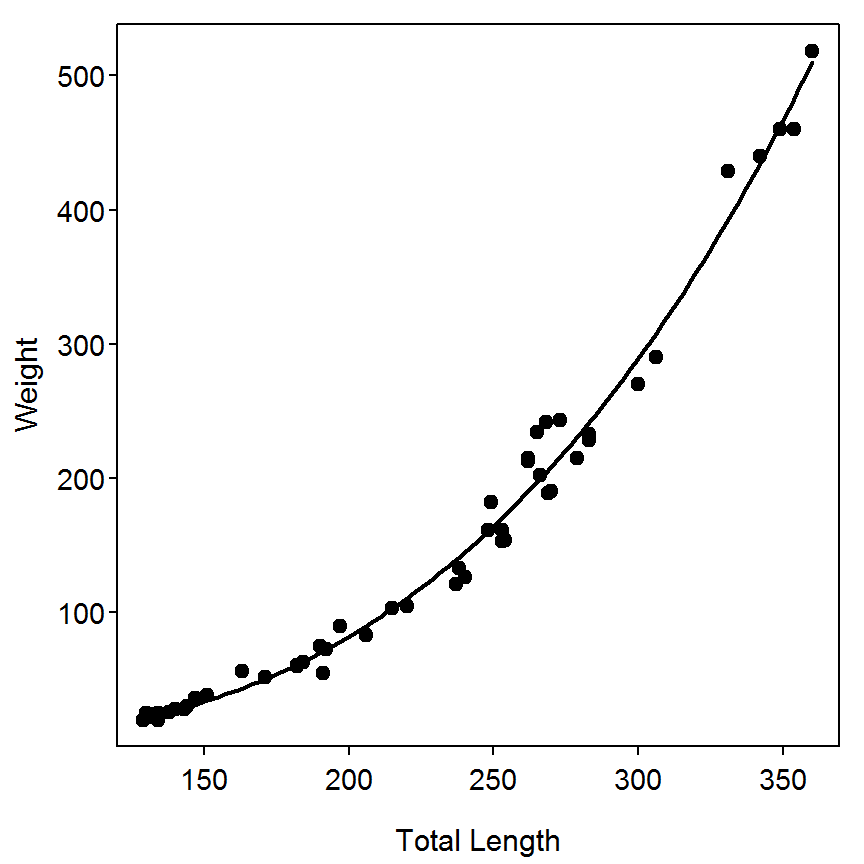``> residPlot(nls1)``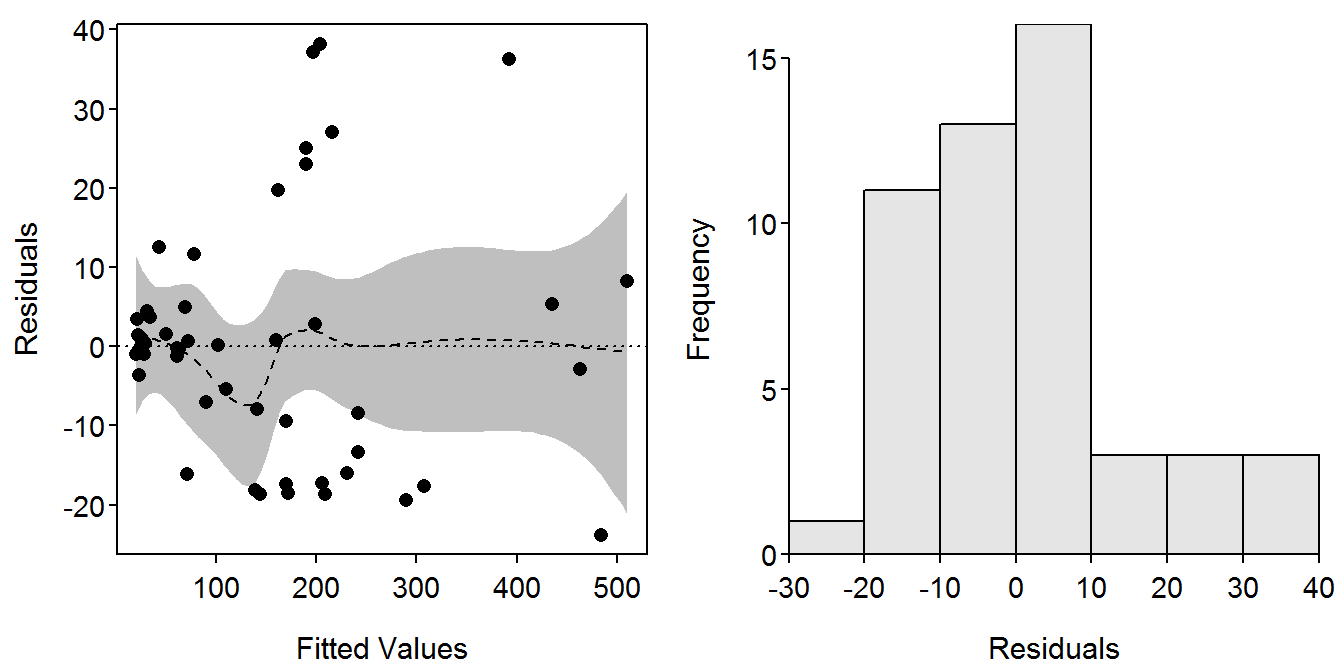Finally, predictions from the non-linear regression model can be obtained with `predict()` as shown above. However, note that intervals can not be computed with `predict()` as shown for the linear model. In general, prediction intervals must be computed with bootstrapping techniques.

``> predict(nls1,data.frame(TL=lens))``
``  164.2616  289.3606 1019.3055``

## 10.2 Analysis of Covariance (ANCOVA)

The ANCOVA can be used to test for differences between regression parameters (i.e., slopes and intercepts) and is especially appropriate when the length ranges sampled in the populations to be compared are generally unequal.

A rough method for comparing slopes from the linear regression between two groups was described in Box 10.1. A more general and precise method for comparing slopes (and entire regression models) is described in the box and is shown below. This methodology is discussed in more detail in Chapter 5 of the Introductory Fisheries Analysis with R book.

### 10.2.1 Preparing Data

The same basic data used in Box 10.1 is used here. However, the example in the box requires a data frame that has all the fish from both “population A” and “population B.” This is most easily accomplished in this situation by creating a subset of the original data frame excluding the fish from “population C.”

``````> d1AB <- Subset(d1,POP!="C")
> str(d1AB)``````
``````'data.frame':   100 obs. of  6 variables:
\$ POP  : Factor w/ 2 levels "A","B": 1 1 1 1 1 1 1 1 1 1 ...
\$ TL   : int  129 130 132 132 134 134 138 140 143 144 ...
\$ WT   : int  20 25 22 24 20 25 26 28 28 30 ...
\$ FAT  : num  5.91 12.88 7.67 11.29 2.27 ...
\$ logTL: num  2.11 2.11 2.12 2.12 2.13 ...
\$ logWT: num  1.3 1.4 1.34 1.38 1.3 ...``````

The full model discussed in the box is fit using `lm()` and a formula of the form `response`~`explanatory*factor`. R will interpret this formula as a short-hand notation for a formula of the form `response`~`explanatory`+ `factor`+ `explanatory:factor`, which is prescribed for this analysis. In addition, R knows to create a set of “dummy” or indicator variables from the levels represented in the `POP` variable because `POP` is a factor. An ANOVA table using type-II SS is constructed by including the saved `lm` object into `Anova()` (from the `car` package) with the `type="II"` argument. From this it is seen, with a non-significant interaction term, that there is no significant difference in the slope between the two populations.

``````> lm3 <- lm(logWT~logTL*POP,data=d1AB)
> Anova(lm3,type="II")``````
``````          Sum Sq     Df   F value    Pr(>F)
logTL     10.205  1.000 5520.8679 < 2.2e-16
POP        0.018  1.000    9.6220  0.002525
logTL:POP  0.001  1.000    0.4244  0.516318
Residuals  0.177 96.000
Total     99.000 10.401                    ``````

The reduced model fit in box 10.2 is fit with `lm()` using a modified formula and the ANOVA results are again extracted with `Anova()`. As shown in the box, there appears to be a significant difference in the intercepts between the two populations. The transformed length-weight relationship are parallel but not the same line between the two populations. The model coefficients and confidence intervals from this final model fit are extracted and a graphic of the model fit is constructed as described above.

``````> lm3r <- lm(logWT~logTL+POP,data=d1AB)
> Anova(lm3r,type="II")``````
``````          Sum Sq     Df   F value    Pr(>F)
logTL     10.205  1.000 5553.8258 < 2.2e-16
POP        0.018  1.000    9.6794  0.002448
Residuals  0.178 97.000
Total     99.000 10.401                    ``````
``> summary(lm3r)``
``````Coefficients:
Estimate Std. Error t value Pr(>|t|)
(Intercept) -5.17144    0.09656 -53.554  < 2e-16
logTL        3.08037    0.04133  74.524  < 2e-16
POPB        -0.03832    0.01232  -3.111  0.00245

Residual standard error: 0.04286 on 97 degrees of freedom
Multiple R-squared: 0.9911, Adjusted R-squared: 0.9909
F-statistic:  5398 on 2 and 97 DF,  p-value: < 2.2e-16 ``````
``> confint(lm3r)``
``````                  2.5 %      97.5 %
(Intercept) -5.36309510 -4.97978797
logTL        2.99833164  3.16240437
POPB        -0.06276474 -0.01387422``````
``> fitPlot(lm3r,xlab="log10 Total Length",ylab="log10 Weight",main="",legend="topleft")``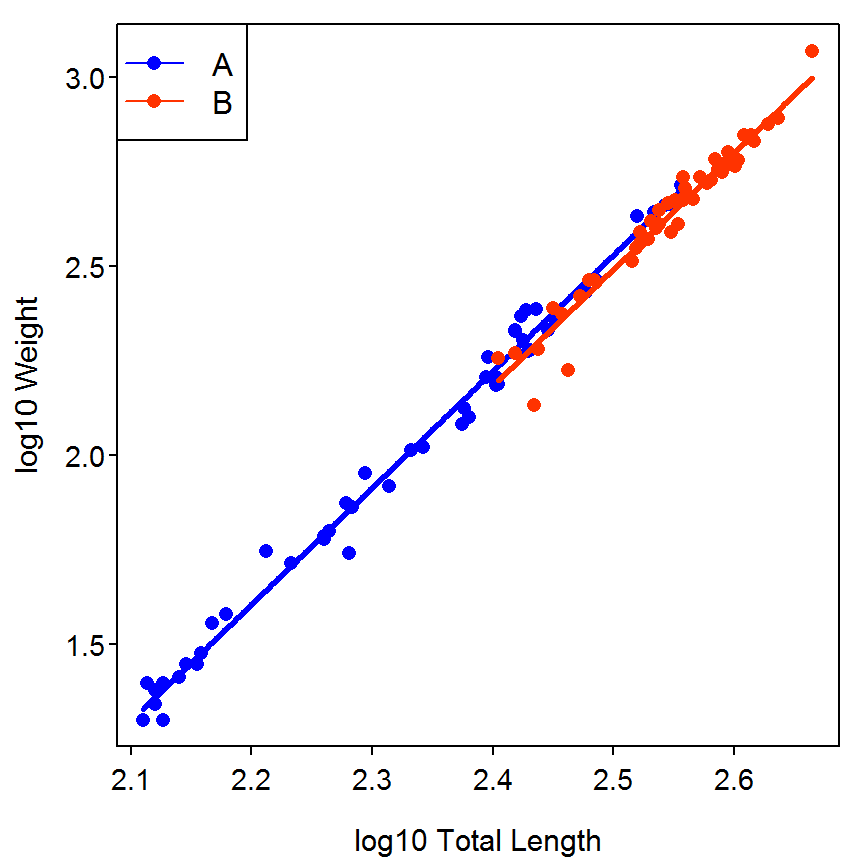## 10.3 Comparisons of Mean Relative Weight

Mean comparison tests such as the two-sample t-test (or the non-parametric equivalent, Mann-Whitney test) or multiple-comparison tests such as ANOVA (or the non parametric Kruskal-Wallis test) can be used to examine length-related or inter-population trends in Wr (relative weight). Herein, we discuss how one might test for difference in condition, as indexed by Wr, among length-groups from the same population or among populations. For the Yellowstone Cutthroat Trout (Oncorhynchus clarkia) data presented in Box 10.1 the question of interest is whether macro-scale habitat type (stream versus lake and low versus high elevation) has any significant influence on fish condition.

Relative weights were calculated for the three populations described in Box 10.1 based on the lotic and length standard-weight equations for Cutthroat Trout (Kruse and Hubert 1997). An important first step is to assess the distribution of the Wr data to determine whether a parametric or non parametric test is more appropriate. This can be completed with typical assessments of normality, such as a histogram or box-plot of the data (not shown). In this case, the data appears generally normal, but there is some skewness and outliers for all three populations. It is important to assess whether the outliers (or individuals with extreme values when compared to the mean) are biologically relevant or errors due to measurement or data entry. We retained the outliers in this assessment.

Prior to comparing overall population means, it is prudent to check for length-related patterns in condition within each population [Murphy et al. (1990); Murphyetal1991; Blackwelletal2000]. For example, changes in Wr with increasing length for Cutthroat Trout from the low-elevation stream population can be assessed by grouping Cutthroat Trout in 50-mm length categories (e.g., group one is 100-149 mm fish and group five is 300-349 mm fish plus the two largest fish). Another way to group the fish is to use the five-cell model (Gabelhouse 1984) for stock-to-trophy length fish (see Cutthroat Trout length categories in Anderson and Neumann (1996)). The following R code calculates Wr values for individual fish and assigns each fish to a length-group for testing differences in Wr among length-groups by means of ANOVA, a test that is robust to small departures from normality.

### 10.3.1 Preparing Data

The same data used in Box 10.1 and Box 10.2 is used here and will not be re-read. However, standard weight and relative weight variables must be added to the data frame. This is made slightly more difficult in this example because the standard weight equation for Cutthroat Trout differs depending on whether it is a lotic or lentic population. In this example, fish in “population A” are lotic and fish in the other two populations are lentic. Thus, I initially created a standard weight variable with no data in it and then populated that variable based on which population the fish was in.

``````> d1\$WS <- as.numeric(NA)
> d1\$WS[d1\$POP=="A"] <- 10^(-5.189+3.099*d1\$logTL[d1\$POP=="A"])  # Lotic
> d1\$WS[d1\$POP!="A"] <- 10^(-5.192+3.086*d1\$logTL[d1\$POP!="A"])  # Lentic
> d1\$WR <- (d1\$WT/d1\$WS)*100
> str(d1)``````
``````'data.frame':   150 obs. of  8 variables:
\$ POP  : Factor w/ 3 levels "A","B","C": 1 1 1 1 1 1 1 1 1 1 ...
\$ TL   : int  129 130 132 132 134 134 138 140 143 144 ...
\$ WT   : int  20 25 22 24 20 25 26 28 28 30 ...
\$ FAT  : num  5.91 12.88 7.67 11.29 2.27 ...
\$ logTL: num  2.11 2.11 2.12 2.12 2.13 ...
\$ logWT: num  1.3 1.4 1.34 1.38 1.3 ...
\$ WS   : num  22.5 23 24.1 24.1 25.3 ...
\$ WR   : num  89 108.6 91.2 99.4 79.1 ...``````

The authors also created a `GRP` variable based on the total length of the fish. This variable can be created with a series of if-then statements as the authors did in the box. However, it is more convenient to use `lencat()`, from the `FSA` package. This function requires a formula of the form ~`len` where `len` is the generic length variable as the first argument and the corresponding data frame in `data=`. There are two ways to use arguments to control how the categories are constructed. First, a starting value can be given in the `startcat=` argument and a constant width of the categories given in the `w=` argument. Alternatively, a sequence of lower boundaries for the categories can be given in the `breaks=` argument. The first method cannot be used in this example because the authors’ last group is wider than the first four groups. The results of `lencat()` should be saved into a data frame which, by default, will have a new variable called `LCat` (the name of variable can be modified by including a new name in the `vname=` argument.

``````> d1 <- lencat(~TL,data=d1,breaks=c(100,150,200,250,300),vname="GRP")
> levels(d1\$GRP)``````
``NULL``
``> headtail(d1)``
``````    POP  TL  WT   FAT    logTL    logWT        WS        WR GRP
1     A 129  20  5.91 2.110590 1.301030  22.47592  88.98411 100
2     A 130  25 12.88 2.113943 1.397940  23.02027 108.59993 100
3     A 132  22  7.67 2.120574 1.342423  24.13563  91.15155 100
148   C 290 231  4.80 2.462398 2.363612 255.24675  90.50066 250
149   C 290 231  4.81 2.462398 2.363612 255.24675  90.50066 250
150   C 290 240  5.74 2.462398 2.380211 255.24675  94.02666 250``````

Finally, the authors focused part of their analysis on the Cutthroat Trout from “population A.” To facilitate this analyses in R I created a new data frame using `Subset()` that contained just the fish from this population (note that this subset was created in BOX 10.1 but need to be re-created here so that it had the new variables just created).

``````> d1A <- Subset(d1,POP=="A")
> str(d1A)``````
``````'data.frame':   50 obs. of  9 variables:
\$ POP  : Factor w/ 1 level "A": 1 1 1 1 1 1 1 1 1 1 ...
\$ TL   : int  129 130 132 132 134 134 138 140 143 144 ...
\$ WT   : int  20 25 22 24 20 25 26 28 28 30 ...
\$ FAT  : num  5.91 12.88 7.67 11.29 2.27 ...
\$ logTL: num  2.11 2.11 2.12 2.12 2.13 ...
\$ logWT: num  1.3 1.4 1.34 1.38 1.3 ...
\$ WS   : num  22.5 23 24.1 24.1 25.3 ...
\$ WR   : num  89 108.6 91.2 99.4 79.1 ...
\$ GRP  : num  100 100 100 100 100 100 100 100 100 100 ...``````

### 10.3.2 One-Way ANOVA Comparison Between Length Groups

The one-way ANOVA model is fit in R with `lm()` using a formula of the form `response`~`factor`. The ANOVA table, summary table, and a plot of the means are constructed by submitting the saved `lm` object to `anova()`, `summary()`, and `fitPlot()`, respectively, as described previously.

``````> lm1 <- lm(WR~GRP,data=d1A)
> anova(lm1)``````
``````          Df Sum Sq Mean Sq F value Pr(>F)
GRP        1   29.6  29.597  0.3257 0.5709
Residuals 48 4361.9  90.872
Total     49 4391.4                       ``````
``> summary(lm1)``
``````Coefficients:
Estimate Std. Error t value Pr(>|t|)
(Intercept) 96.85175    4.05113  23.907   <2e-16
GRP         -0.01112    0.01949  -0.571    0.571

Residual standard error: 9.533 on 48 degrees of freedom
Multiple R-squared: 0.00674,    Adjusted R-squared: -0.01395
F-statistic: 0.3257 on 1 and 48 DF,  p-value: 0.5709 ``````
``> fitPlot(lm1,xlab="Total Length Category",ylab="Relative Weight",main="")``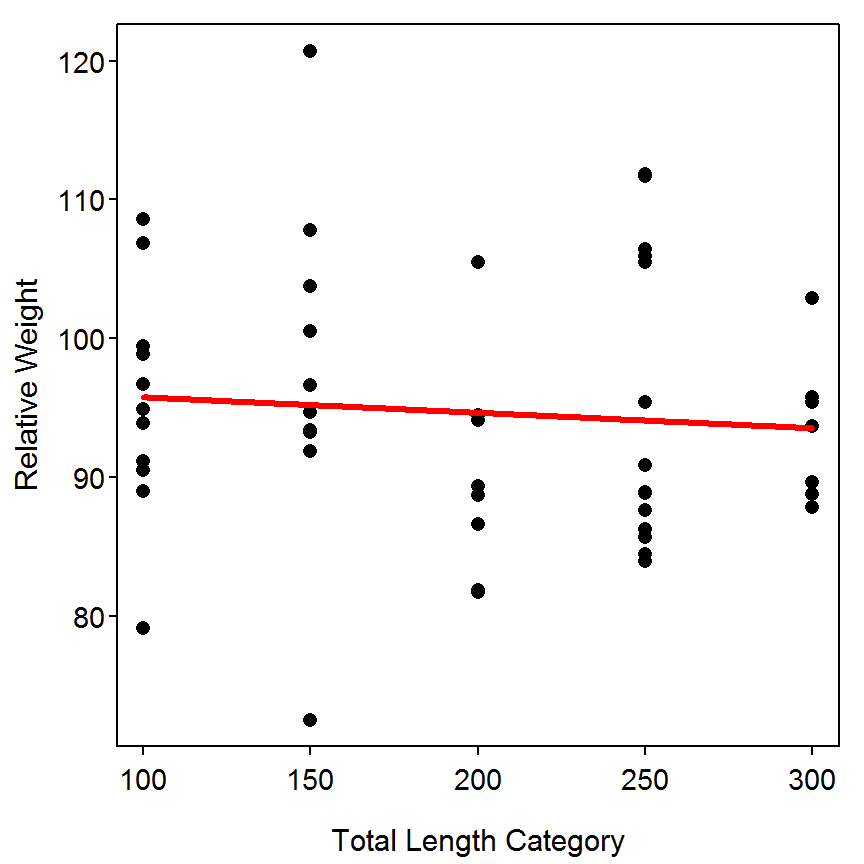A residual plot for assessing linearity and homoscedasticity and a histogram of residuals for assessing normality are constructed with `residPlot()` as described previously.

``> residPlot(lm1)``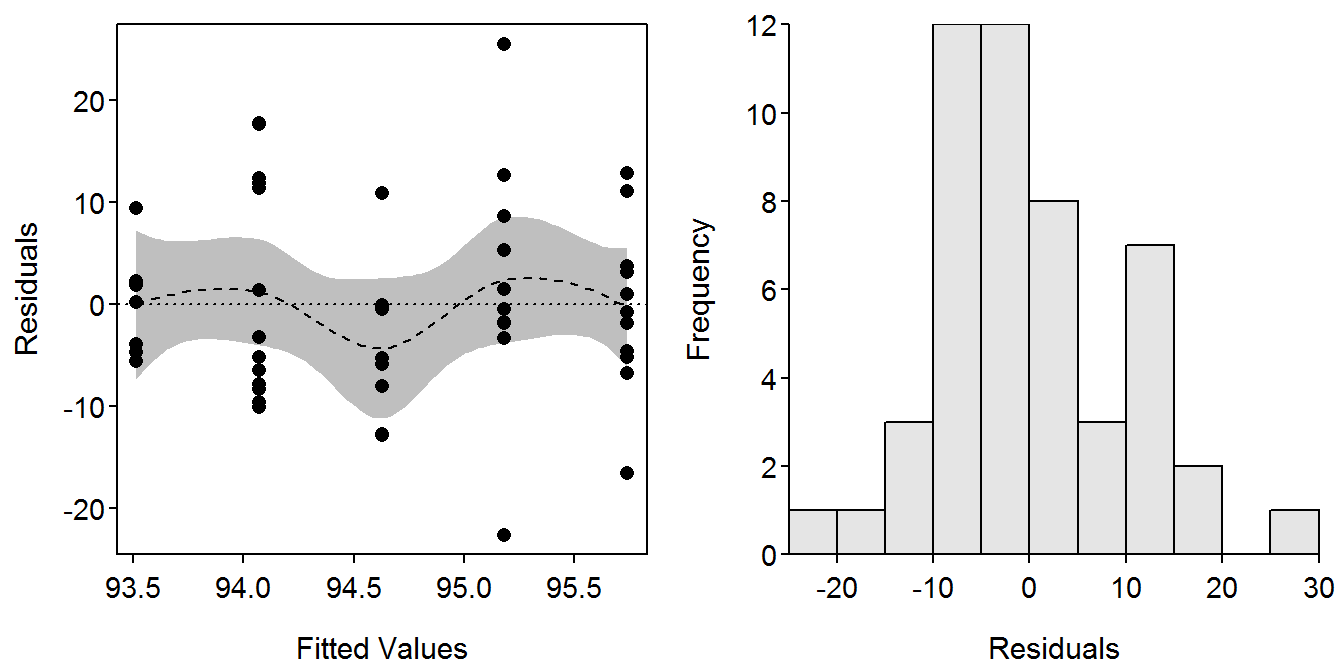### 10.3.3 Kruskal-Wallis Test Comparison Between Length Groups

A non-parametric Kruskal-Wallis test to compare the median relative weight between length groups is conducted with `kruskal.test()`, which requires the same to arguments as used in `lm()`.

``> kruskal.test(WR~GRP,data=d1A)``
``````Kruskal-Wallis rank sum test with WR by GRP
Kruskal-Wallis chi-squared = 4.138, df = 4, p-value = 0.3877``````

### 10.3.4 One-Way ANOVA Comparison Among Populations

The one-way ANOVA for comparing mean relative weight among the three populations is performed similarly.

``````> lm2 <- lm(WR~POP,data=d1)
> anova(lm2)``````
``````           Df  Sum Sq Mean Sq F value Pr(>F)
POP         2   276.4 138.197  1.7507 0.1773
Residuals 147 11604.1  78.939
Total     149 11880.5                       ``````
``> summary(lm2)``
``````Coefficients:
Estimate Std. Error t value Pr(>|t|)
(Intercept)   94.672      1.256  75.346   <2e-16
POPB          -1.384      1.777  -0.779   0.4372
POPC          -3.310      1.777  -1.863   0.0645

Residual standard error: 8.885 on 147 degrees of freedom
Multiple R-squared: 0.02326,    Adjusted R-squared: 0.009976
F-statistic: 1.751 on 2 and 147 DF,  p-value: 0.1773 ``````
``> Summarize(WR~POP,data=d1,digits=2)``
``````  POP  n  mean   sd   min    Q1 median     Q3   max percZero
1   A 50 94.67 9.47 72.52 88.77  93.76 100.20 120.7        0
2   B 50 93.29 8.46 64.93 89.22  93.33  99.21 108.9        0
3   C 50 91.36 8.70 67.19 88.14  90.88  96.57 109.5        0``````
``> fitPlot(lm2,xlab="Population",ylab="Relative Weight",main="")``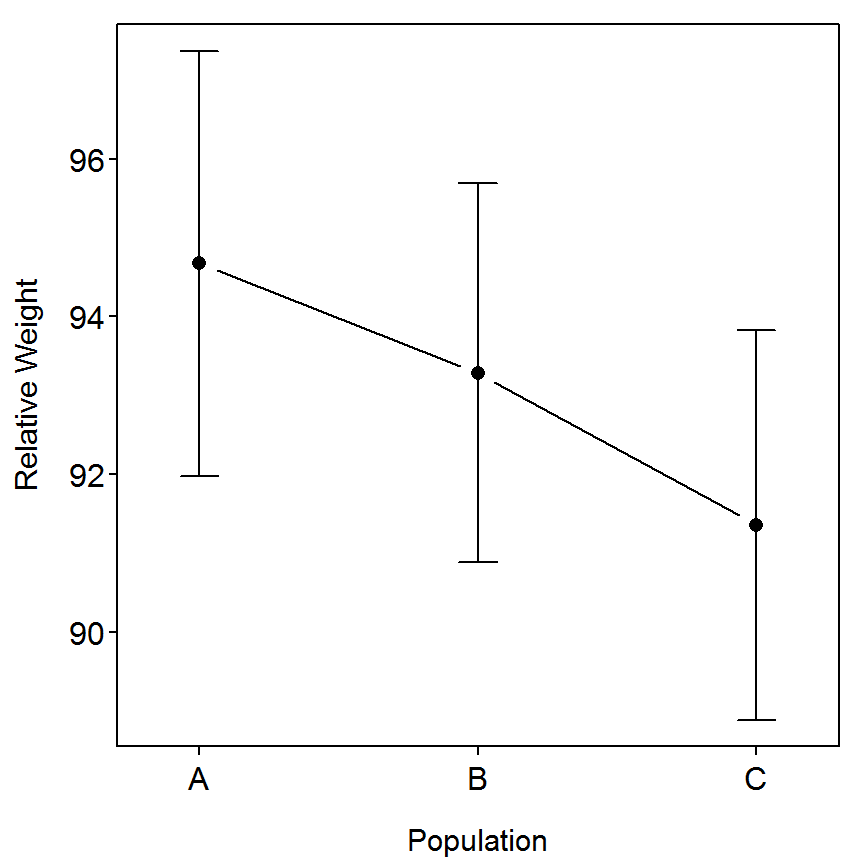``> residPlot(lm2)``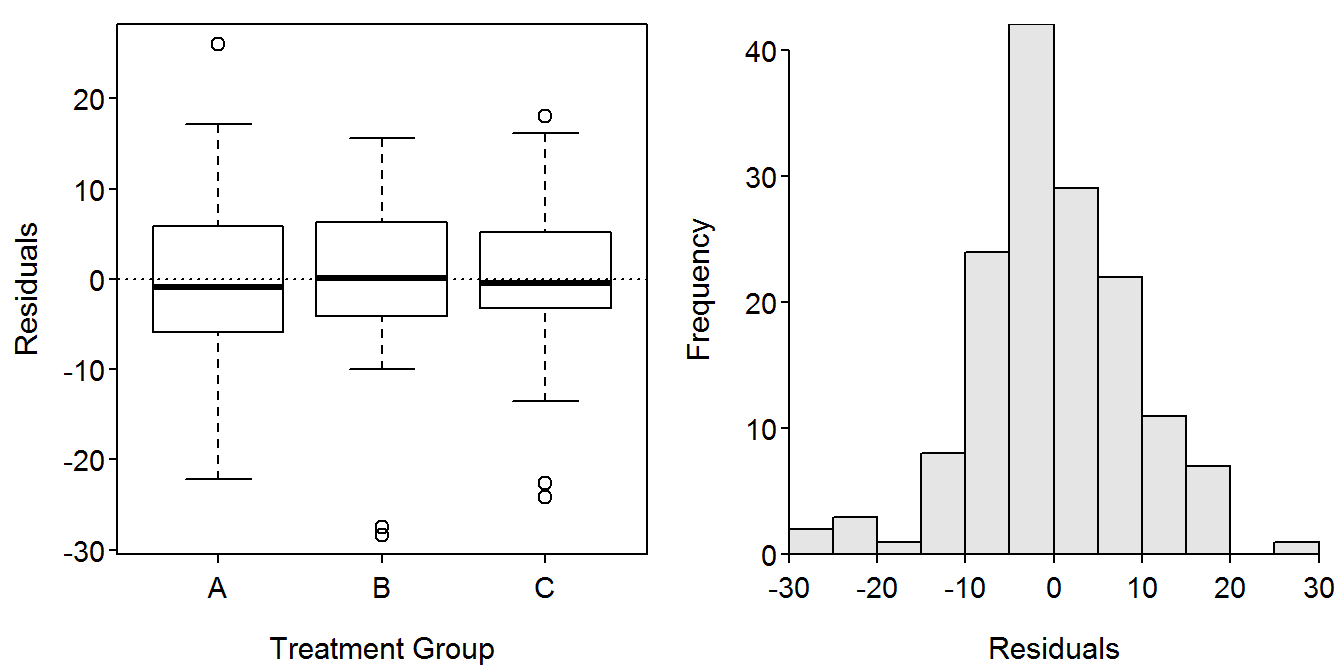## 10.4 Analysis of Fat Composition Data

In Box 10.2, we tested for differences in Wr within and among populations. Here we examine whether those Wr values are related to whole-body fat content in individual fish and then test whether population mean fat content differs among populations. Fat composition, a direct measure of individual wellness or energy stores, was estimated for the Yellowstone Cutthroat Trout sampled in stream and lake habitats (see Box 10.1 for data). We compared fat composition to Wr by means of correlation and regression analyses. The question of interest is whether Wr is a good indicator of individual physiological fitness as referenced by tissue fat content. Additionally, we want to know if using fat as the indicator of individual fitness results in a different conclusion regarding the population-level effects that elevation (a surrogate for environmental conditions such as temperature, growing season, and food supply) might have on fish condition. Please note that in this example we did not check for length-related biases (e.g., potential differences among length categories) within each population. The following R code regresses wet weight fat percentage against individual Wr (all populations combined into one data set) and compares mean percent fat composition among the three Yellowstone Cutthroat Trout populations by means of ANOVA.

### 10.4.1 Comparing Relative Weight to Fat Composition

The linear regression is fit and the ANOVA and summary tables, summary graphic, residual plots, and histogram of residuals are constructed as described previously.

``````> lm3 <- lm(FAT~WR,data=d1)
> anova(lm3)``````
``````           Df  Sum Sq Mean Sq F value    Pr(>F)
WR          1  857.46  857.46  158.72 < 2.2e-16
Residuals 148  799.54    5.40
Total     149 1657.00                          ``````
``> summary(lm3)``
``````Coefficients:
Estimate Std. Error t value Pr(>|t|)
(Intercept) -18.44302    1.99447  -9.247 2.37e-16
WR            0.26865    0.02132  12.599  < 2e-16

Residual standard error: 2.324 on 148 degrees of freedom
Multiple R-squared: 0.5175, Adjusted R-squared: 0.5142
F-statistic: 158.7 on 1 and 148 DF,  p-value: < 2.2e-16 ``````
``> fitPlot(lm3,xlab="Relative Weight",ylab="Fat Composition",main="")``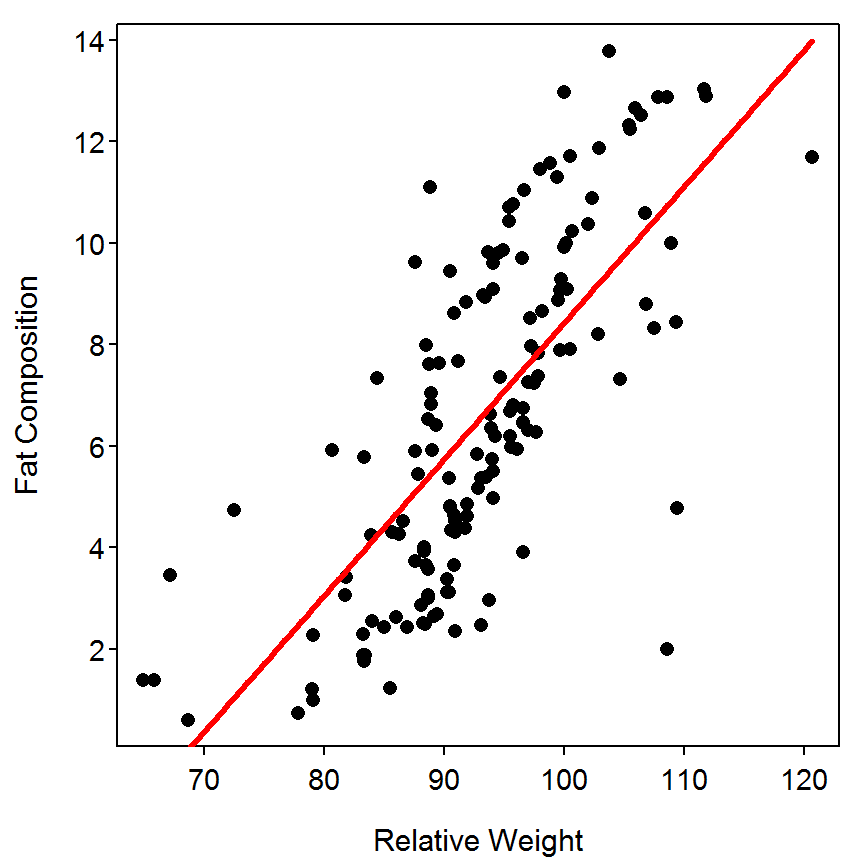``> residPlot(lm3)``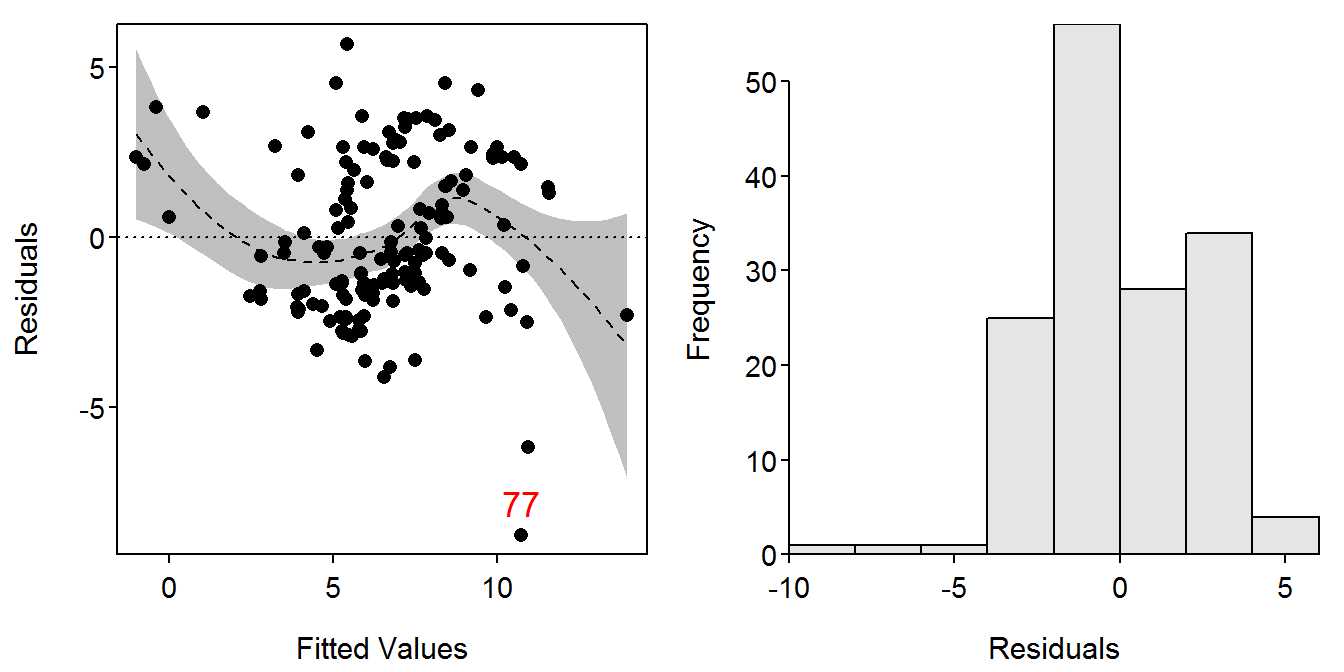### 10.4.2 Testing Fat Composition Differences Among Populations

The one-way ANOVA and results are fit as described previously.

``````> lm4 <- lm(FAT~POP,data=d1)
> anova(lm4)``````
``````           Df  Sum Sq Mean Sq F value    Pr(>F)
POP         2  414.86  207.43  24.548 6.329e-10
Residuals 147 1242.14    8.45
Total     149 1657.00                          ``````
``> Summarize(FAT~POP,data=d1,digits=2)``
``````  POP  n mean   sd  min   Q1 median    Q3   max percZero
1   A 50 8.84 3.08 2.27 6.55   9.04 11.50 13.78        0
2   B 50 5.98 3.13 1.38 3.07   5.81  8.82 12.98        0
3   C 50 4.90 2.46 0.60 3.00   4.79  6.41 10.89        0``````
``> residPlot(lm4)``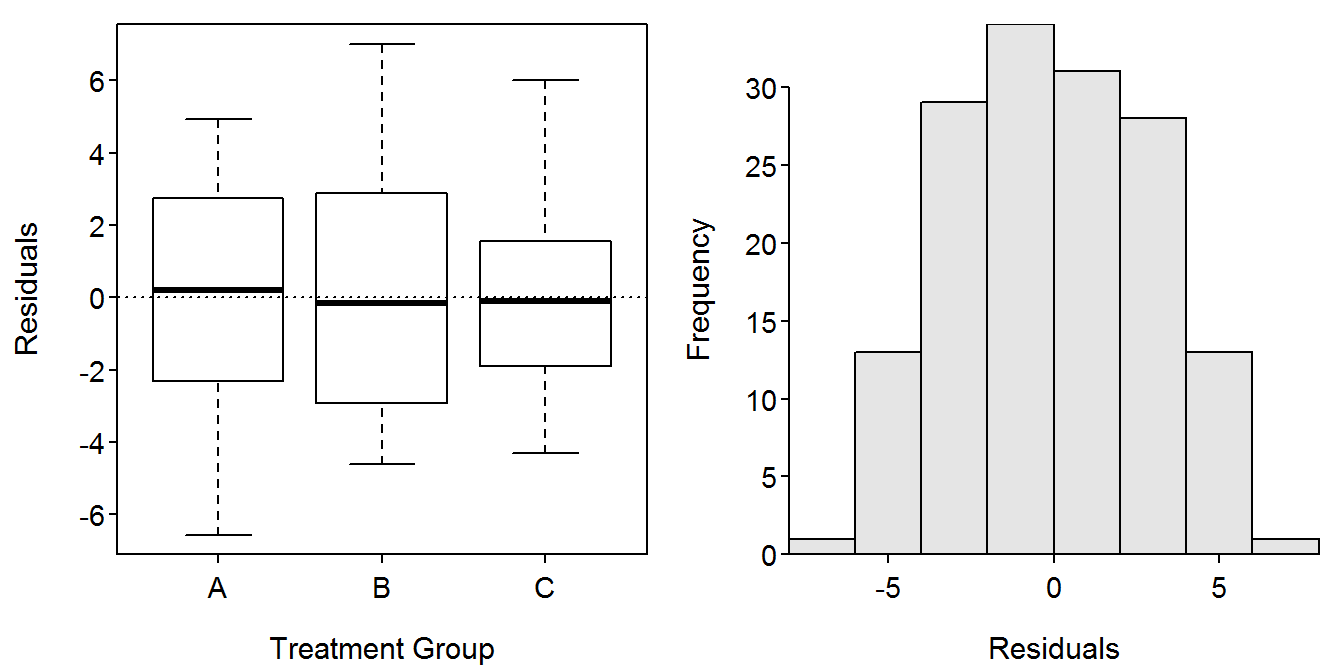The Tukey multiple comparisons results are obtained by submitting the `lm` object as the first argument to `glht()`, from the `multcomp` package. This function requires a second argument that indicates which type of multiple comparison procedure to use. This second argument uses `mcp()` which requires the factor variable set equal to the word “Tukey” to perform the Tukey multiple comparison procedure. The saved `glht` object is submitted to `summary()` to get the difference in means with a corresponding hypothesis test p-value among each pair of groups and to `confint()` to get the corresponding confidence intervals for the difference in means. In addition, submitting the saved `glht` object to `cld()` will produce “significance letters” to indicate which means are different (different letters mean different means).

``````> mc1 <- glht(lm4,mcp(POP="Tukey"))
> summary(mc1)``````
``````           Estimate Std. Error t value Pr(>|t|)
B - A == 0  -2.8594     0.5814  -4.918   <1e-04
C - A == 0  -3.9424     0.5814  -6.781   <1e-04
C - B == 0  -1.0830     0.5814  -1.863    0.153``````
``> confint(mc1)``
``````           Estimate lwr     upr
B - A == 0 -2.8594  -4.2359 -1.4829
C - A == 0 -3.9424  -5.3189 -2.5659
C - B == 0 -1.0830  -2.4595  0.2935``````
``> cld(mc1)``
``````  A   B   C
"b" "a" "a" ``````

A graphic of the model results is obtained with `fitPlot()` (as noted above) and the significance letters are placed on the means plot with `addSigLetters()` (`addSigLetters()` is from the `FSA` package. You should examine the help for this function to see what each of the arguments is used for).

``````> fitPlot(lm4,xlab="Population",ylab="Fat Composition",main="")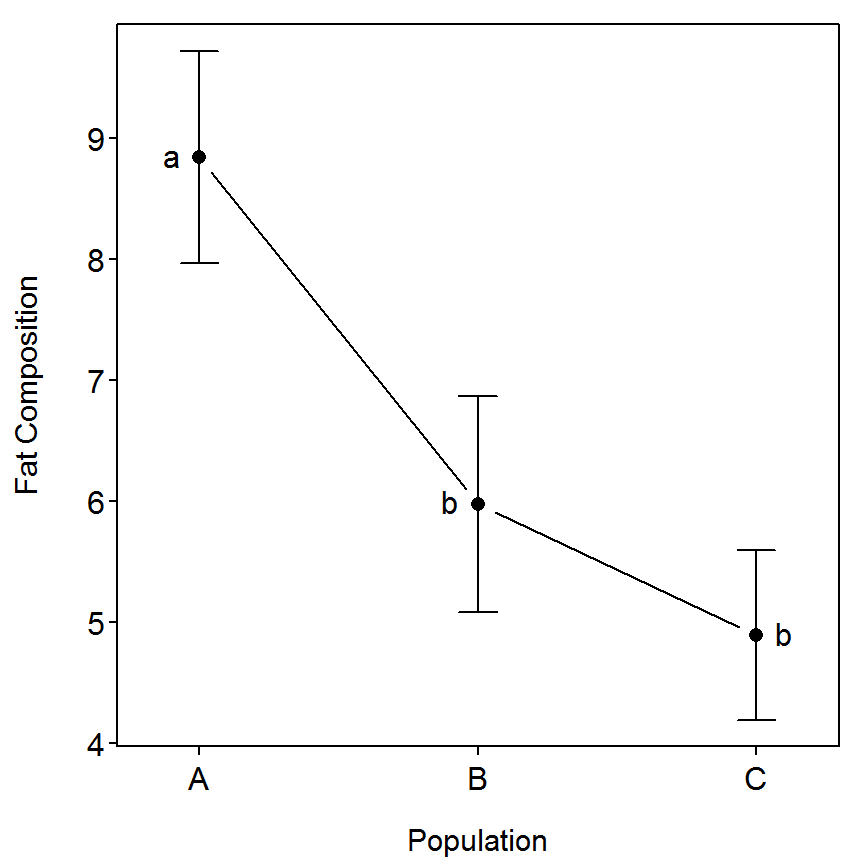## 10.5 Morphological Assessment of Juvenile Condition

The following data are used to assess effects of starvation on body condition of largemouth bass (Micropterus salmoides) juveniles. For most fishes, standard condition indices (e.g., Wr) are applicable to only adults and large juveniles because weight measures are imprecise for small fish. A controlled experiment was conducted to determine if simple morphological measurements could be used to determine condition of juvenile largemouth bass (partial data set from Smith et al. (2005)). Hatchery-reared largemouth bass were raised until completion of fin development and then divided into two experimental groups of fed and unfed fish. Differences in body morphology existed after only 3 d (days) of food deprivation, and a simple bivariate ratio of body depth at the anus to standard length was almost as efficient and robust at classifying fed and unfed largemouth bass as a multivariate index based on 23 morphometric characters. Here we provide an assessment of differences in the body depth after 6 d (days) of food deprivation.

### 10.5.1 Preparing Data

The Box10_5.txt data file is read and the structure of the data frame is observed.

``````> d <- read.table("data/Box10_5.txt",header=TRUE)
> str(d)``````
``````'data.frame':   52 obs. of  3 variables:
\$ food: Factor w/ 2 levels "Fed","Unfed": 1 1 1 1 1 1 1 1 1 1 ...
\$ SL  : num  9.24 9.27 9.5 9.29 9.29 ...
\$ BD  : num  1.71 1.73 1.9 1.81 1.81 ...``````

### 10.5.2 Comparison of Regressions Between Groups

The full model discussed in the box is fit using `lm()` as described previously. An ANOVA table using type-II SS is constructed by including the saved `lm` object to `Anova()` (from the `car` package) with the `type="II"` argument. From this it is seen, with a non-significant interaction term, that there is no significant difference in the slope between the two groups.

``````> lm1 <- lm(BD~SL*food,data=d)
> Anova(lm1,type="II")``````
``````          Sum Sq      Df  F value    Pr(>F)
SL         3.914  1.0000 212.5614 < 2.2e-16
food       0.618  1.0000  33.5542 5.192e-07
SL:food    0.000  1.0000   0.0042    0.9488
Residuals  0.884 48.0000
Total     51.000  5.4164                   ``````

The reduced model fit in box 10.5 is fit in R and ANOVA results extracted below. As shown in the box, there appears to be a significant difference in the intercepts between the two populations. Thus the transformed length-weight relationship are parallel but not the same line between the two populations. The model coefficients and confidence intervals from this final model fit are extracted with `summary()` and `confint()`, respectively. The graphic of the model fit is again created with `fitPlot()`.

``````> lm1r <- lm(BD~SL+food,data=d)
> Anova(lm1r,type="II")``````
``````          Sum Sq      Df F value    Pr(>F)
SL         3.914  1.0000  216.97 < 2.2e-16
food       0.618  1.0000   34.25 3.948e-07
Residuals  0.884 49.0000
Total     51.000  5.4164                  ``````
``> summary(lm1r)``
``````Coefficients:
Estimate Std. Error t value Pr(>|t|)
(Intercept) -0.30440    0.16490  -1.846   0.0709
SL           0.23301    0.01582  14.730  < 2e-16
foodUnfed   -0.26277    0.04490  -5.852 3.95e-07

Residual standard error: 0.1343 on 49 degrees of freedom
Multiple R-squared: 0.8213, Adjusted R-squared: 0.814
F-statistic: 112.6 on 2 and 49 DF,  p-value: < 2.2e-16 ``````
``> confint(lm1r)``
``````                 2.5 %      97.5 %
(Intercept) -0.6357708  0.02697857
SL           0.2012207  0.26479883
foodUnfed   -0.3530021 -0.17254185``````
``> fitPlot(lm1r,xlab="Standard Length (mm)",ylab="Body Depth (mm)",main="",legend="topleft")``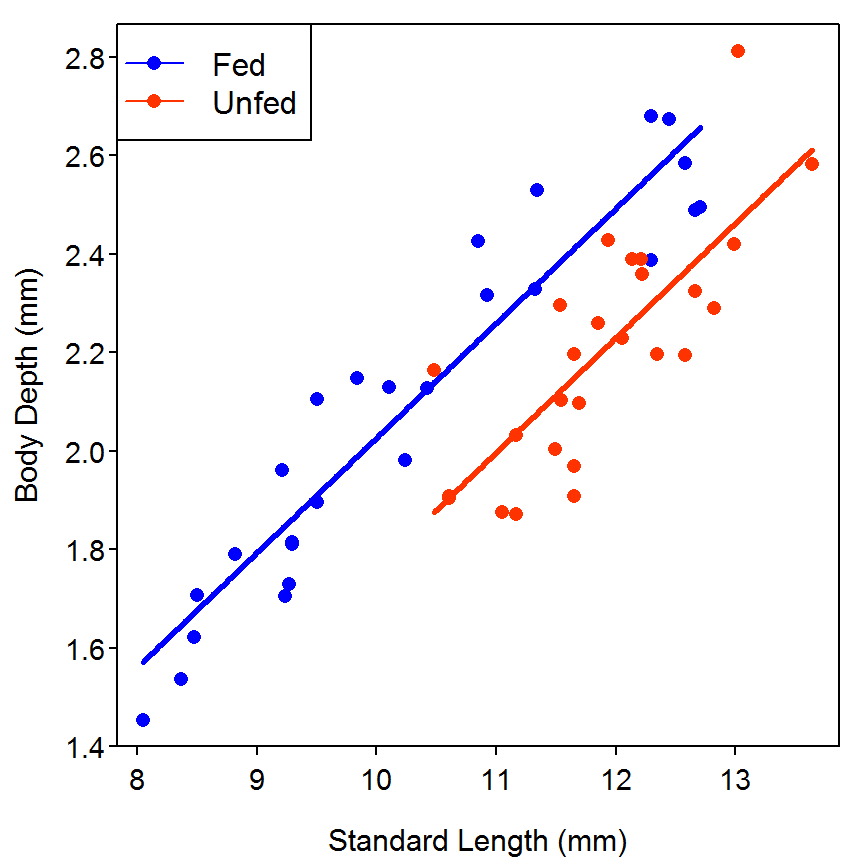The model diagnostics are constructed as shown above.

``> residPlot(lm1r)``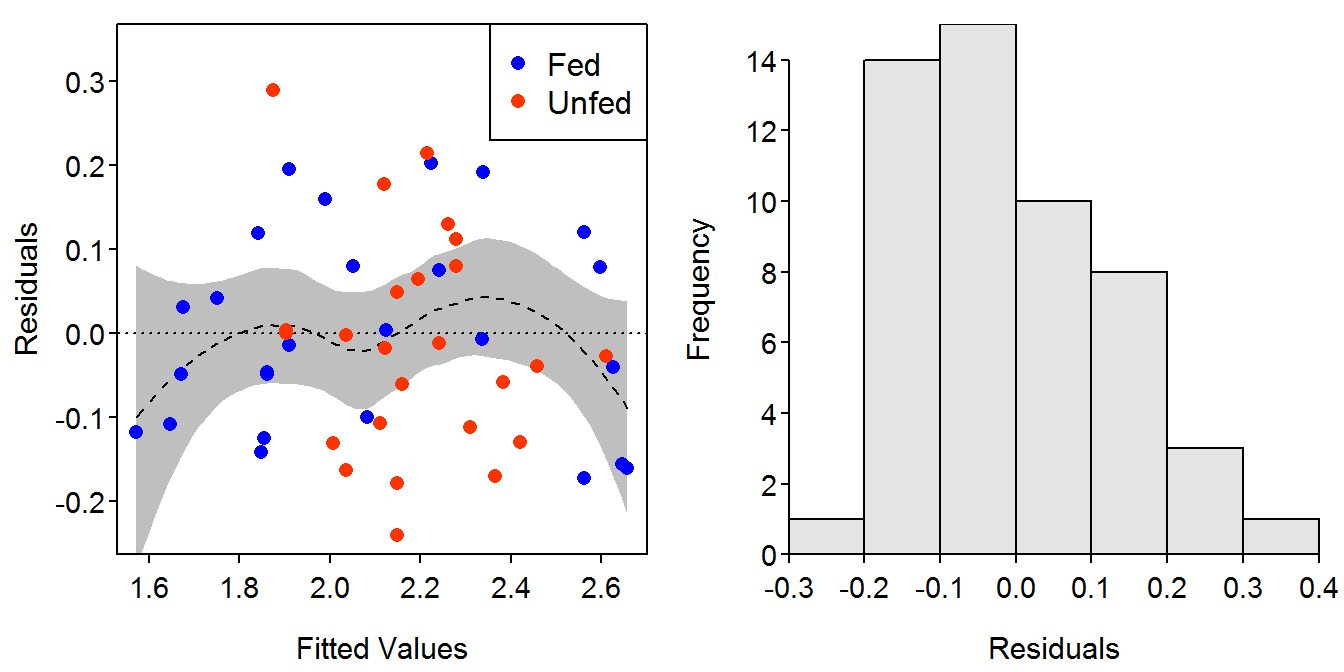## 10.6 Use of Fulton’s Condition to Assess the Effects of Parasites

Parasites may negatively affect the condition of fish. Here we determine if condition of Arkansas River shiners (Notropis girardi) (29-60 mm TL) is reduced when fish are parasitized by anchor worm, a cosmopolitan cyclopoid copepod. Arkansas River shiners were captured with a seine (see Hayes et al. (1996) for a discussion of this gear), measured (`TL`; mm), weighed (0.1 g), and inspected to determine the presence of the parasite (partial data set from Durham et al. (2002)). Differences in condition among fish with and without the parasite were assessed using ANOVA to test differences in Fulton’s condition (K), an appropriate assessment metric as the fish are from a single population over identical size ranges. Individual fish from this experiment were treated as the experimental unit because our research questions asked if differences in condition existed between two populations of Arkansas River shiners (population contained parasites (`with`) and population contained no parasites(`without`)).

### 10.6.1 Preparing Data

The Box10_6.txt data file is read, the structure of the data frame is observed, and a random six rows are observed. Log-transformed versions of the `TL` and `WT` variables and a variable containing Fulton’s condition factor for each fish were appended to the data frame. Note that the data is organized here in a stacked format rather than the unstacked format presented in the box.

``````> d6 <- read.table("data/Box10_6.txt",header=TRUE)
> str(d6)``````
``````'data.frame':   60 obs. of  3 variables:
\$ Lernaea: Factor w/ 2 levels "with","without": 1 1 1 1 1 1 1 1 1 1 ...
\$ TL     : int  29 29 34 35 38 38 39 39 40 42 ...
\$ WT     : num  0.21 0.187 0.286 0.356 0.42 0.46 0.448 0.252 0.514 0.555 ...``````
``````> d6\$logTL <- log10(d6\$TL)
> d6\$logWT <- log10(d6\$WT)
> d6\$K <- d6\$WT/(d6\$TL^3)*10000   # Fulton's condition factor
> headtail(d6)                        # six random rows from the data frame``````
``````   Lernaea TL    WT    logTL      logWT          K
1     with 29 0.210 1.462398 -0.6777807 0.08610439
2     with 29 0.187 1.462398 -0.7281584 0.07667391
3     with 34 0.286 1.531479 -0.5436340 0.07276613
58 without 52 1.273 1.716003  0.1048284 0.09053539
59 without 57 1.573 1.755875  0.1967287 0.08493842
60 without 60 1.686 1.778151  0.2268576 0.07805556``````

The authors perform separate regressions for the shiners with and without the parasite. To facilitate these analyses in R I created new data frames using `Subset()` that will separately contain just the fish from the two groups based on the presence of the parasite.

``````> d6w <- Subset(d6,Lernaea=="with")
> str(d6w)``````
``````'data.frame':   30 obs. of  6 variables:
\$ Lernaea: Factor w/ 1 level "with": 1 1 1 1 1 1 1 1 1 1 ...
\$ TL     : int  29 29 34 35 38 38 39 39 40 42 ...
\$ WT     : num  0.21 0.187 0.286 0.356 0.42 0.46 0.448 0.252 0.514 0.555 ...
\$ logTL  : num  1.46 1.46 1.53 1.54 1.58 ...
\$ logWT  : num  -0.678 -0.728 -0.544 -0.449 -0.377 ...
\$ K      : num  0.0861 0.0767 0.0728 0.083 0.0765 ...``````
``````> d6wo <- Subset(d6,Lernaea=="without")
> str(d6wo)``````
``````'data.frame':   30 obs. of  6 variables:
\$ Lernaea: Factor w/ 1 level "without": 1 1 1 1 1 1 1 1 1 1 ...
\$ TL     : int  29 31 31 31 31 32 33 35 36 39 ...
\$ WT     : num  0.175 0.254 0.228 0.201 0.219 0.269 0.278 0.356 0.356 0.478 ...
\$ logTL  : num  1.46 1.49 1.49 1.49 1.49 ...
\$ logWT  : num  -0.757 -0.595 -0.642 -0.697 -0.66 ...
\$ K      : num  0.0718 0.0853 0.0765 0.0675 0.0735 ...``````

### 10.6.2 Summary Statistics for Both Groups

The summary statistics presented in the box are computed with `Summarize()`, from the `FSA` package. In this example, this function requires, as the first argument, a formula of the form `quantitative~factor` where `quantitative` is the generic representation of the quantitative variable and `factor` is the generic representation of the group classification or factor variable. The second argument – i.e., `data=` argument – contains a data frame which holds the quantitative and factor variables.

``> Summarize(TL~Lernaea,data=d6)``
``````  Lernaea  n     mean       sd min    Q1 median    Q3 max percZero
1    with 30 44.83333 7.579843  29 39.25   45.5 49.00  60        0
2 without 30 42.60000 8.536332  29 35.25   44.0 48.75  60        0``````
``> Summarize(WT~Lernaea,data=d6)``
``````  Lernaea  n      mean        sd   min    Q1 median     Q3   max percZero
1    with 30 0.6856667 0.3100578 0.187 0.451  0.660 0.8805 1.388        0
2 without 30 0.7008000 0.4093965 0.175 0.356  0.632 0.9745 1.686        0``````
``> Summarize(K~Lernaea,data=d6)``
``````  Lernaea  n      mean        sd     min      Q1  median      Q3     max
1    with 30 0.0719516 0.0121006 0.04248 0.06380 0.07421 0.08090 0.09323
2 without 30 0.0802046 0.0056098 0.06747 0.07688 0.08018 0.08368 0.09437
percZero
1        0
2        0``````

### 10.6.3 Separate Length-Weight Regressions

The two regressions demonstrated in the box are constructed with `lm()` followed by the use of `anova()`, `summary()`, and `confint()`, as illustrated above.

``````> lmw <- lm(logWT~logTL,data=d6w)
> anova(lmw)``````
``````          Df  Sum Sq Mean Sq F value    Pr(>F)
logTL      1 1.29841 1.29841   214.9 1.157e-14
Residuals 28 0.16917 0.00604
Total     29 1.46758                          ``````
``> summary(lmw)``
``````Coefficients:
Estimate Std. Error t value Pr(>|t|)
(Intercept)  -4.6945     0.3060  -15.34 3.70e-15
logTL         2.7234     0.1858   14.66 1.16e-14

Residual standard error: 0.07773 on 28 degrees of freedom
Multiple R-squared: 0.8847, Adjusted R-squared: 0.8806
F-statistic: 214.9 on 1 and 28 DF,  p-value: 1.157e-14 ``````
``> confint(lmw)``
``````                2.5 %    97.5 %
(Intercept) -5.321228 -4.067769
logTL        2.342846  3.103935``````
``````> lmwo <- lm(logWT~logTL,data=d6wo)
> anova(lmwo)``````
``````          Df  Sum Sq Mean Sq F value    Pr(>F)
logTL      1 2.27177 2.27177  2782.1 < 2.2e-16
Residuals 28 0.02286 0.00082
Total     29 2.29463                          ``````
``> summary(lmwo)                                                                                                        ``
``````Coefficients:
Estimate Std. Error t value Pr(>|t|)
(Intercept) -5.30889    0.09634  -55.10   <2e-16
logTL        3.13084    0.05936   52.75   <2e-16

Residual standard error: 0.02858 on 28 degrees of freedom
Multiple R-squared:  0.99,  Adjusted R-squared: 0.9897
F-statistic:  2782 on 1 and 28 DF,  p-value: < 2.2e-16 ``````
``> confint(lmwo)``
``````                2.5 %    97.5 %
(Intercept) -5.506232 -5.111540
logTL        3.009256  3.252432``````

### 10.6.4 Comparing Length-Weight Regressions

The authors of the box did not compare, for example, the log weight and log total length regression between groups with or without the anchor worms. However, this is accomplished simply with a formula in `lm()` modified for an ANCOVA (as described previously). The significant interaction term (\(p=0.0322\)) indicates that the slope of the log weight to log total length regression differs significantly between the two groups. The fitted line plot indicated that the rate of increase of log weight for increasing log total length is greater when the anchor worms are not present then when they are present.

``````> lmc <- lm(logWT~logTL*Lernaea,data=d6)
> Anova(lmc,type="II")``````
``````              Sum Sq      Df   F value    Pr(>F)
logTL          3.554  1.0000 1036.2810 < 2.2e-16
Lernaea        0.039  1.0000   11.4161  0.001331
logTL:Lernaea  0.017  1.0000    4.8283  0.032150
Residuals      0.192 56.0000
Total         59.000  3.8014                    ``````
``> summary(lmc)``
``````Coefficients:
Estimate Std. Error t value Pr(>|t|)
(Intercept)           -4.6945     0.2305 -20.366   <2e-16
logTL                  2.7234     0.1400  19.458   <2e-16
Lernaeawithout        -0.6144     0.3035  -2.024   0.0477
logTL:Lernaeawithout   0.4075     0.1854   2.197   0.0322

Residual standard error: 0.05856 on 56 degrees of freedom
Multiple R-squared: 0.949,  Adjusted R-squared: 0.9463
F-statistic: 347.7 on 3 and 56 DF,  p-value: < 2.2e-16 ``````
``> fitPlot(lmc,xlab="log10 Total Length",ylab="log10 Weight",main="",legend="topleft")``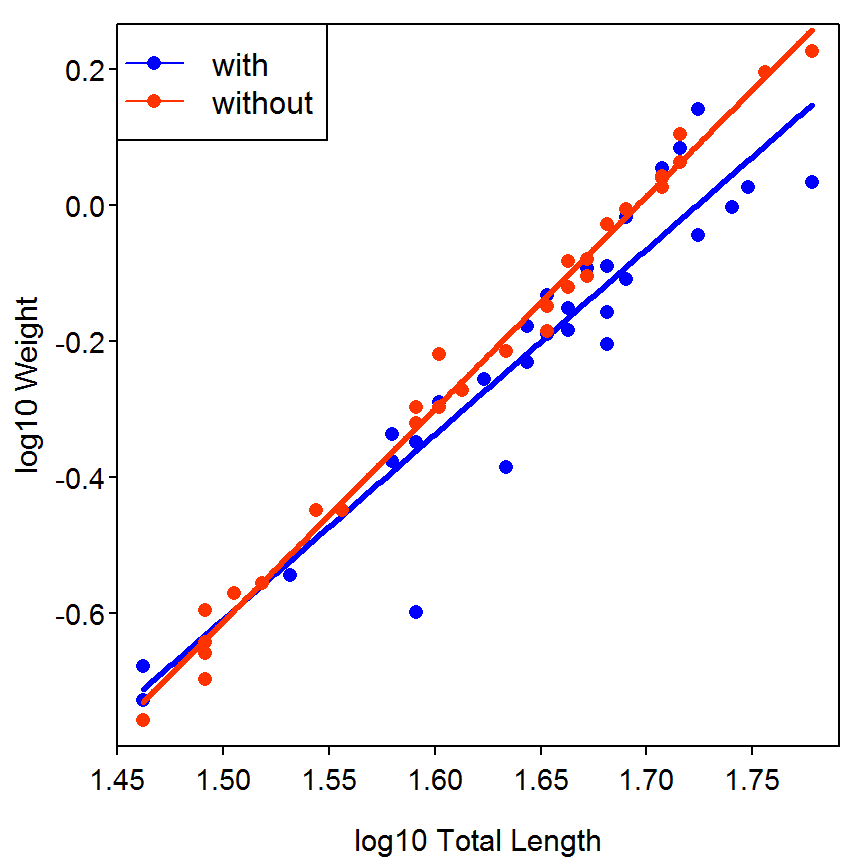A residual plot suggests a potential outlier in the data set and a possible slight heteroscedasticity in the group with anchor worms.

``> residPlot(lmc,main="")``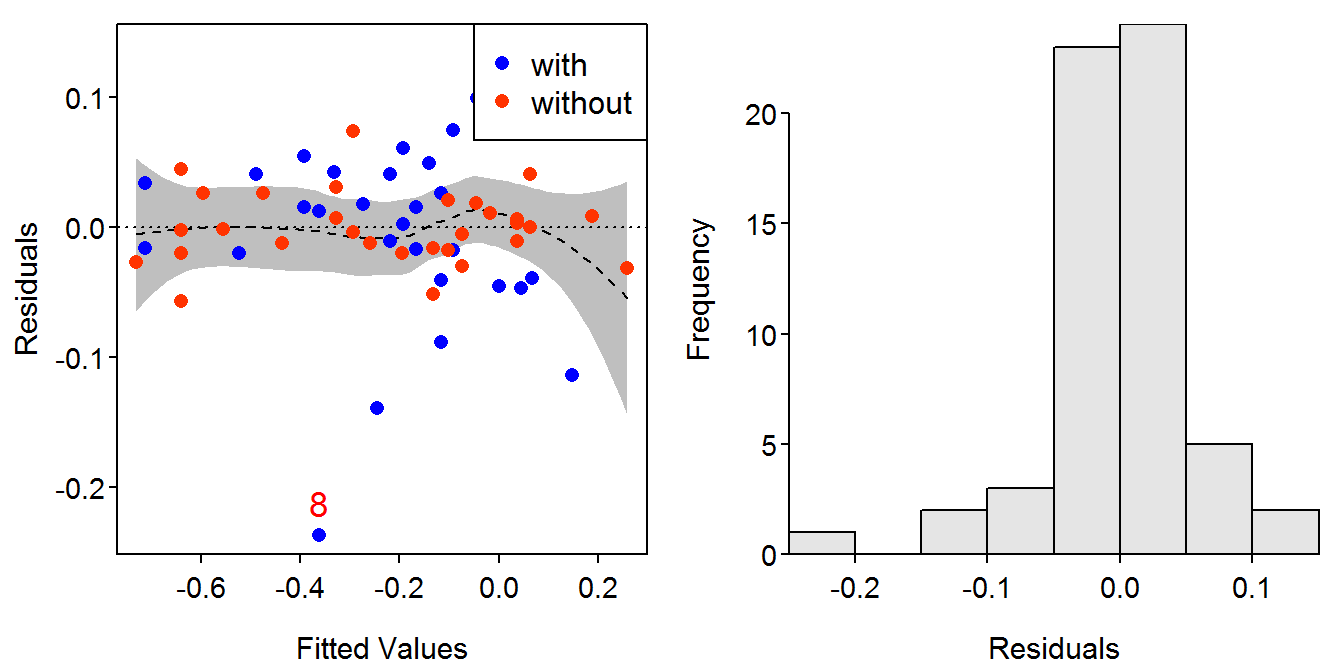### 10.6.5 Separate One-Way ANOVAs

The separate ANOVAs demonstrated in the box are computed below.

``````> lmTL <- lm(TL~Lernaea,data=d6)
> anova(lmTL)``````
``````          Df Sum Sq Mean Sq F value Pr(>F)
Lernaea    1   74.8  74.817  1.1482 0.2884
Residuals 58 3779.4  65.161
Total     59 3854.2                       ``````
``> summary(lmTL)``
``````Coefficients:
Estimate Std. Error t value Pr(>|t|)
(Intercept)      44.833      1.474  30.420   <2e-16
Lernaeawithout   -2.233      2.084  -1.072    0.288

Residual standard error: 8.072 on 58 degrees of freedom
Multiple R-squared: 0.01941,    Adjusted R-squared: 0.002505
F-statistic: 1.148 on 1 and 58 DF,  p-value: 0.2884 ``````
``````> lmWT <- lm(WT~Lernaea,data=d6)
> anova(lmWT)``````
``````          Df Sum Sq  Mean Sq F value Pr(>F)
Lernaea    1 0.0034 0.003435  0.0261 0.8723
Residuals 58 7.6485 0.131871
Total     59 7.6519                        ``````
``> summary(lmWT)``
``````Coefficients:
Estimate Std. Error t value Pr(>|t|)
(Intercept)     0.68567    0.06630  10.342  8.8e-15
Lernaeawithout  0.01513    0.09376   0.161    0.872

Residual standard error: 0.3631 on 58 degrees of freedom
Multiple R-squared: 0.0004489,  Adjusted R-squared: -0.01678
F-statistic: 0.02605 on 1 and 58 DF,  p-value: 0.8723 ``````
``````> lmK <- lm(K~Lernaea,data=d6)
> anova(lmK)``````
``````          Df    Sum Sq    Mean Sq F value   Pr(>F)
Lernaea    1 0.0010217 0.00102168  11.486 0.001266
Residuals 58 0.0051589 0.00008895
Total     59 0.0061806                            ``````
``> summary(lmK)``
``````Coefficients:
Estimate Std. Error t value Pr(>|t|)
(Intercept)    0.071952   0.001722  41.786  < 2e-16
Lernaeawithout 0.008253   0.002435   3.389  0.00127

Residual standard error: 0.009431 on 58 degrees of freedom
Multiple R-squared: 0.1653, Adjusted R-squared: 0.1509
F-statistic: 11.49 on 1 and 58 DF,  p-value: 0.001266 ``````

### 10.6.6 Visualizations I

The plots depicted on the last page of the box are constructed by first plotting the points for only when the parasite is present using `plot()` and then adding the points for when the parasite was not present with `points()`.

``````> plot(WT~TL,data=d6,subset=Lernaea=="with",ylab="Wt(g)",xlab="TL (mm)",pch=16)
> points(WT~TL,data=d6,subset=Lernaea=="without",pch=1)
> legend("topleft",legend=c("Lernaea present","Lernaea absent"),pch=c(16,1),cex=0.8)``````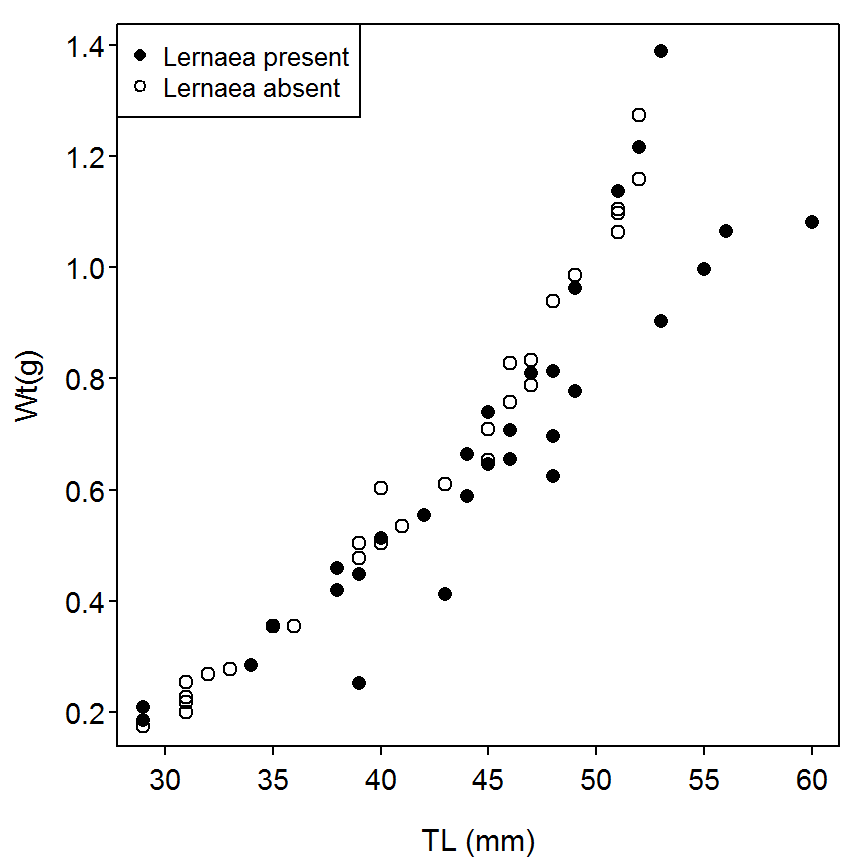``````> plot(logWT~logTL,data=d6,subset=Lernaea=="with",ylab="log10(Wt)",xlab="log10(TL)",pch=16)
> points(logWT~logTL,data=d6,subset=Lernaea=="without",pch=1)``````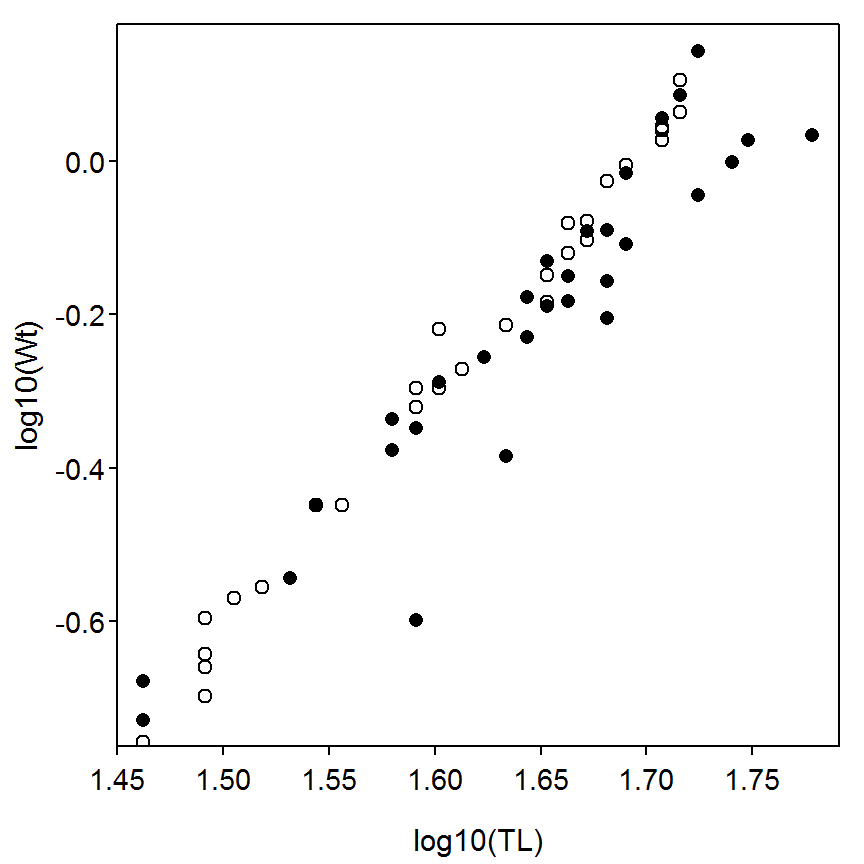``````> plot(K~TL,data=d6,subset=Lernaea=="with",ylab="K",xlab="TL (mm)",pch=16)
> points(K~TL,data=d6,subset=Lernaea=="without",pch=1)``````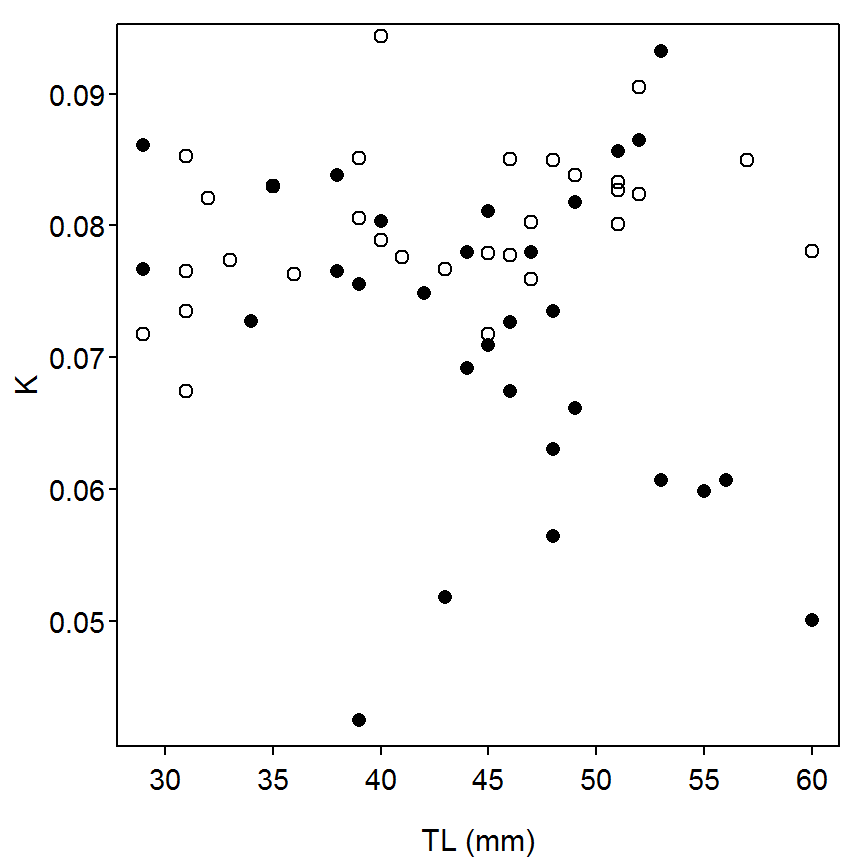### 10.6.7 Visualizations II

The graphics depicted below use `xyplot()`, from the `Lattice` package.

``> xyplot(WT~TL,group=Lernaea,data=d6,xlab="Total Length (mm)",ylab="Weight(g)",auto.key=TRUE)``